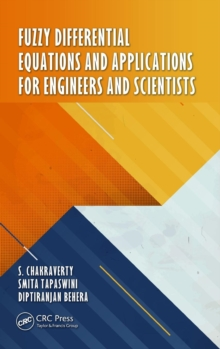Supporting your high street Find out how »
• My Account# Fuzzy Differential Equations and Applications for Engineers and Scientists Hardback

#### Description

Differential equations play a vital role in the modeling of physical and engineering problems, such as those in solid and fluid mechanics, viscoelasticity, biology, physics, and many other areas.

In general, the parameters, variables and initial conditions within a model are considered as being defined exactly.

In reality there may be only vague, imprecise or incomplete information about the variables and parameters available.

This can result from errors in measurement, observation, or experimental data; application of different operating conditions; or maintenance induced errors.

To overcome uncertainties or lack of precision, one can use a fuzzy environment in parameters, variables and initial conditions in place of exact (fixed) ones, by turning general differential equations into Fuzzy Differential Equations ("FDEs").

In real applications it can be complicated to obtain exact solution of fuzzy differential equations due to complexities in fuzzy arithmetic, creating the need for use of reliable and efficient numerical techniques in the solution of fuzzy differential equations.

These include fuzzy ordinary and partial, fuzzy linear and nonlinear, and fuzzy arbitrary order differential equations. This unique work provides a new direction for the reader in the use of basic concepts of fuzzy differential equations, solutions and its applications.

It can serve as an essential reference work for students, scholars, practitioners, researchers and academicians in engineering and science who need to model uncertain physical problems.

#### Information

• Format: Hardback
• Pages: 224 pages, 3 Line drawings, black and white; 120 Halftones, black and white; 53 Tables, black and wh
• Publisher: Apple Academic Press Inc.
• Publication Date:
• Category: Differential calculus & equations
• ISBN: 9781482244731

£110.00

£101.19

on all orders

###### Pick up orders

from local bookshops